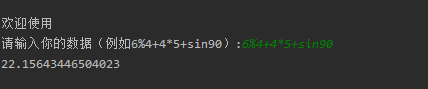# 20182204 实验二《Python程序设计》实验报告

## 1.实验内容

• 设计并完成一个完整的应用程序，完成加减乘除模等运算，功能多多益善。
• 考核基本语法、判定语句、循环语句、逻辑运算等知识点

## 2. 实验过程及结果

"""
文件名：Python测试
作者：20182204赵文普
时间：3.25
"""
import math

print("\n欢迎使用")
s = input("请输入你的数据（例如6%4+4*5+sin90）:")
i = 0

digit = []
char = []
for c in s:
if c.isdigit():
digit.append(c)
else:
char += c

def multiplication(k):
digit[k] = int(digit[k]) * int(digit[k + 1])

def division(k):
digit[k] = int(digit[k]) / int(digit[k + 1])

def mod(k):
digit[k] = int(digit[k]) % int(digit[k + 1])

def sin(k):
b = float(digit[k]) / 180 * math.pi
digit[k] = math.sin(b)

def cos(k):
b = float(digit[k]) / 180 * math.pi
digit[k] = math.cos(b)

def tan(k):
b = float(digit[k]) / 180 * math.pi
digit[k] = math.tan(b)

number1 = 0
k = 0
while k < len(char):
if char[k] == "*":
multiplication(k)
elif char[k] == "/":
division(k)
elif char[k] == "%":
mod(k)
elif char[k] == "s":
sin(k)
elif char[k] == "c":
cos(k)
elif char[k] == "t":
tan(k)
k += 1
k = 0
result = float(digit)
while k < len(char):
if char[k] == "+":
result += float(digit[k + 1])
elif char[k] == "-":
result -= float(digit[k + 1])
k += 1
print(result)## 3. 实验过程中遇到的问题和解决过程

• 问题1：数学方面根号、三角函数不知如何表示。
• 问题1解决方案：通过百度查到引入import math：math.sqrt() 、 math.sin()、math.pi等
• 问题2：如何判断用户输入的是字符还是数字
• 问题2解决方案：百度后查明引用c.isdigit 完成了输入

## 感悟

posted @ 2020-04-11 23:44  182204  阅读(112)  评论(0编辑  收藏